## 23 December 2019

### 620. Divide into two parts

 Easy Medium Hard Extreme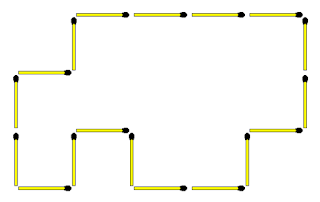Add 3 matchsticks to the layout above to divide it into 2 parts equal in shape and size.

## 23 March 2019

### 619. Mathematical: 7 minus 2 is not 8

 Easy Medium Hard Extreme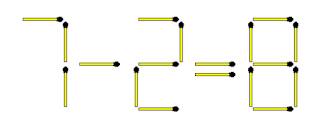TAKE AWAY 2 matchsticks to make the equation true.

## 15 February 2019

### 618. Mathematical: 4 minus 0 is not 5

 Easy Medium Hard Extreme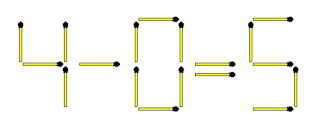ADD 3 matchsticks to make the equation true. Negative numbers are not allowed and no number is greater than 9.

## 02 February 2019

### 617. Change the matchstick heads

 Easy Medium Hard ExtremeThere are 4 vertical-, 4 horizontal- and 2 diagonal rows (diagonally from outer corner to corner) in the layout above. The matchstick heads in each row are encircled.

Rearrange the matchsticks to end with 6 matchstick heads in each row.

## 20 January 2019

### 616. Five to three squares

 Easy Medium Hard Extreme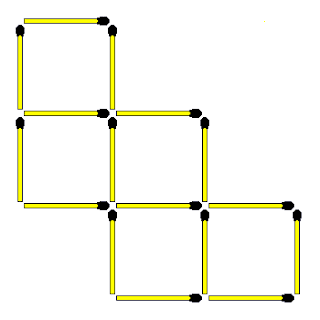Move 4 matchsticks to create 3 squares. The squares may differ in size.

## 06 January 2019

### 615. Mathematical: 2 minus 2 is not 5

 Easy Medium Hard ExtremeADD 3 matchsticks to make the equation true. Negative numbers are not allowed and no number is greater than 9.

## 28 December 2018

### 614. Divide the shape

 Easy Medium Hard Extreme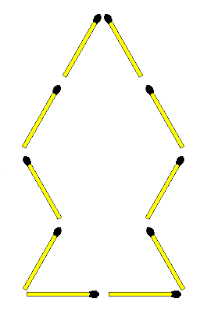Add TWO matchsticks to the layout above to divide the area into two parts. The two parts must be equal in size and shape.

## 22 December 2018

### 613. Five to six squares

 Easy Medium Hard ExtremeMove 4 matchsticks to create 6 squares. Squares may differ in size.
The four small squares are:
CLFE, LDGF, EFJH, FGKJ

The two big squares are:
ABGE, CDKH

## 15 December 2018

### 612. Ship to Triangles

 Easy Medium Hard ExtremeMOVE four matchsticks to convert the ship into eight triangles.

The triangles may differ in size and all matchsticks don't have to be flat on the surface.
4 big triangles:
ACH, BKD, CEJ, CJH

4 small triangles:
BCF, CDG, FKH, GJK

## 08 December 2018

 Easy Medium Hard Extreme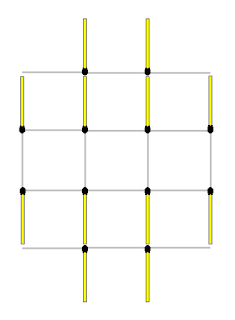If four matchstick heads form the four corners of a square, how many squares can you identify in the layout above? Squares my differ in size.

Be warned, this is not so easy!
There are 11 squares

Small: ABED, CDHG, DEJH, EFKJ, HJML
Medium: CAEH, BFJD, KMHE, LGDJ
Big: CBKL, AFMG

## 01 December 2018

### 610. Mathematical: 9 minus 6 is not 4

 Easy Medium Hard ExtremeMOVE 3 matchsticks to make the equation true.

There are four possible answers. Getting one solution within 30 seconds makes you good. Getting four solutions within three minutes makes you brilliant!

## 24 November 2018

### 609. Five to two squares

 Easy Medium Hard ExtremeThere are 5 equal squares in the layout above. Move 6 matchsticks to reduce the number of squares to two. The two squares MUST be different in size.

## 17 November 2018

### 608. Spot the twins

 Easy Medium Hard Extreme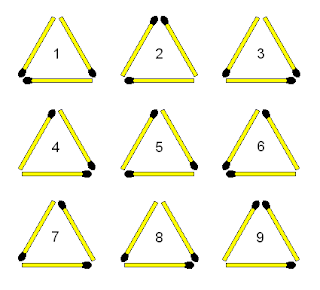How long does it take you to spot the two identical triangles?
Numbers 3 and 8 are identical.

## 12 November 2018

### 607. Mathematical: 4 - 0 is not 7

 Easy Medium Hard Extreme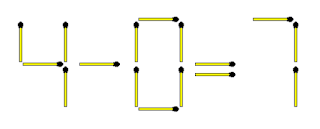ADD 5 matchsticks to make the equation true. No number may be greater than 9 or negative.

## 04 November 2018

### 606. Nodes: 4 matchsticks

 Easy Medium Hard ExtremeThere are 4 nodes in the layout above (the circles) and the node value is 8. A node can therefore be described as the touching point of two or more matchsticks OR the two ends of a matchstick. A matchstick head has a value of 2 and the opposite side a value of 1.

How would you place 4 matchsticks to create a node value of exactly 20?

## 28 October 2018

### 605. Five matchsticks in a row

 Easy Medium Hard ExtremeFive matchsticks are placed with heads pointing North as shown above. The challenge is to turn ALL the matchsticks so that all the heads are pointing South. The following rules apply when turning:

1. Turn 3 matchsticks at a time.
2. The 3 matchsticks you turn don't have to be adjacent.

Can you achieve this in 3 steps?

## 13 October 2018

### 603. Move 3 to create 2 rhombuses

 Easy Medium Hard Extreme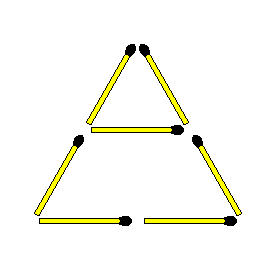Move 3 matchsticks to create 2 rhombuses. (also known as diamonds) The 2 rhombuses must be equal in size.

## 06 October 2018

### 602. Divide into equal parts

 Easy Medium Hard ExtremePlace 7 matchsticks inside the layout above to form 3 equal shapes. The 3 shapes must be equal in shape and size.

## 29 September 2018

### 601. Rectangle 3X2: Remove 6

 Easy Medium Hard Extreme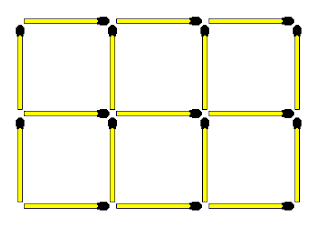TAKE AWAY 6 matchsticks to create 2 squares. The 2 squares must not be equal in size.

## 15 September 2018

### 600. The clock and the number

 Easy Medium Hard ExtremeBeware, another sneaky one!

Where would you ADD one matchstick to make the statement true?

## 08 September 2018

### 599. Mathematical: 3 minus 9 is not 9

 Easy Medium Hard Extreme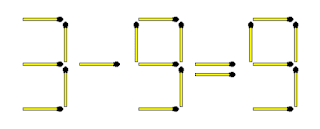TAKE AWAY 5 matchsticks to make the equation true. Can you find both solutions?

## 25 August 2018

### 598. Three squares and four rectangles

 Easy Medium Hard Extreme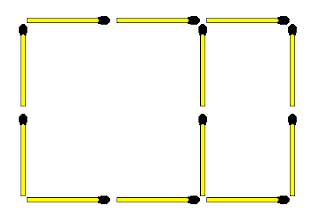There is one square and one rectangle in the layout above. ADD 6 matchsticks to form three equal squares and four equal rectangles.
The four equal rectangles are easy to identify, but the three equal squares are:

ACHK, BDGJ and CEFH

## 15 August 2018

### 597. Not Roman numerals

 Easy Medium Hard Extreme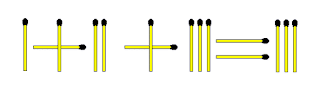Move only ONE matchstick to make the equation true.

## 11 August 2018

### 596. M for Matchstick

 Easy Medium Hard ExtremeAdd 2 matchsticks to form 10 triangles. The matchsticks may overlap and do not have to be flat on the surface.
There are 4 Small triangles:

ALK
BCM
OME
LOJ

There are 6 combined triangles

AJH
BDE
LEF
MJF
KOH
CDO

## 28 July 2018

### 595. Polygon with 8 sides

 Easy Medium Hard ExtremeShown above is a seven sided convex polygon built from 2 squares and 3 triangles. 12 matchsticks were used to achieve this. The outside angles are all greater than 180 degrees as illustrated with angle "a" above.

Create your own convex polygon. The following rules apply:

1. Polygon must have 8 sides
2. 17 matchsticks to be used
3. All outside angles to be greater than 180 degrees
4. There must be 6 equal sized triangles
5. There must be 2 equal sized squares
6. All matchsticks must be flat on the surface.